# The Classical Theory of Interest (With Diagarm)

Read this article to learn about the classical theory of Interest, demand for savings, supply for savings, equilibrium rate of interest and criticism!

The classical theory of interest also known as the demand and supply theory was propounded by the economists like Marshall and Fisher.

Later on, Pigou, Cassel, Knight and Taussig worked to modify the theory.

According to this theory rate of interest is determined by the intersection of demand and supply of savings. It is called the real theory of interest in the sense that it explains the determination of interest by analyzing the real factors like savings and investment. Therefore, classical economists maintained that interest is a price paid for the supply of savings.

### Demand for Savings:

Demand for savings comes from those who want to invest in business activities. Demand for investment is derived demand. Any factor of production is demanded for its productivity. The demand for the factor is high when there are higher expectations from it.Since, all the factors are not equally productive, so, capital demand will be high for more productive uses first and then gradually with the increase in its supply, will shift to less productive uses.

Therefore, classical economists maintained that with the aid of capital facilities we turn out more goods per man-hour than when we produce with bare hands or with scant tools. Moreover, marginal productivity of the business goes on decreasing with more and more doses of investment of savings in his business venture. It is due to the operation of the law of diminishing returns.

Now a very important question arises is that how much capital a person will demand because when a person borrows money he has to pay interest on it. The answer according to this theory is that demand for capital can be raised to a point where marginal productivity of capital becomes equal to the interest paid on it. Thus, if marginal productivity of capital is more than the interest paid, then it is beneficial to borrow money and vice-versa. Equilibrium will prevail at a point where marginal productivity of capital equals the rate of interest. This shows that there exists inverse relationship between demand for capital and the interest rate.

This fact can be made clear with the help of the following table 1 and diagram 1: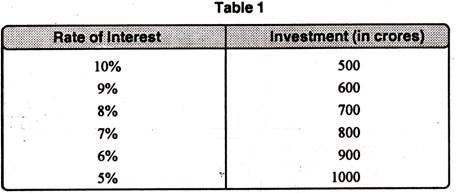Table 1 shows that rate of interest and investment are inversely related to each other. As the rate of interest increases, the level of investment declines and vice-versa. As in the table, initially, the rate of interest is 10%, investment is Rs. 500 crores. When the rate of interest decreases to 8%, the level of investment increases to Rs. 700 crores from Rs. 500 crores. Further, as the rate of interest again falls to 5%, the level of investment increases to Rs. 1000 crores.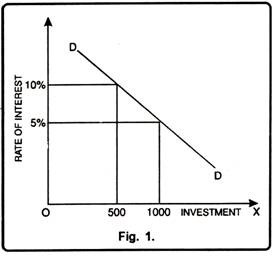The Fig. 1 depicts that there exists inverse relationship between the investment and the rate of interest. Initially, the rate of interest is 10%, the level of investment is Rs. 500 crores. Now the rate of interest falls to 5%. With this decrease in the interest rate, level of investment increases to Rs. 1000 crores. It indicates that more capital is demanded at a low interest rate and vice versa.

### Supply of Savings:

Supply of capital is the result of savings. It comes from those who have the excess of income over consumption. Thus, savings is the main source of capital which depends on the capacity to save, willingness to save, level of income and rate of interest etc. Capacity to save depends on the size of national income, size of personal income, size of family, price level and purchasing power of money etc. Willingness to save depends on the family affection, further expectations etc.

To a large extent, willingness to save is affected by the rate of interest. On a higher rate of interest people save more to earn the benefits of high rate of interest. On the other hand, at the low rate of interest, people save less. Thus, we may say that there is a direct relationship between the supply of savings and the rate of interest. The following table and diagram justifies this fact in a more vivid way.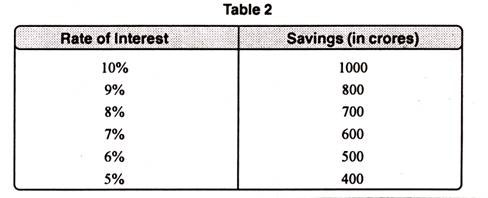It is clear from the table 2 that rate of interest and savings have a positive relationship. As the rate of interest increases, savings will also increase. On the other hand, a fall in rate of interest leads to a decrease in savings. When the rate of interest is 10%, the savings are of Rs. 1000 crores.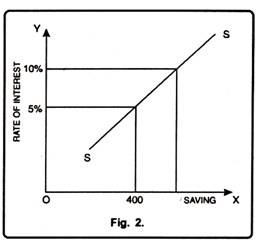In the successive periods, as rate of interest falls from 10% to 5%, the total savings also decline. Suppose as the rate of interest falls to 5%, savings also decrease to Rs. 400 crores.

In Fig. 2 savings have been represented on X-axis and interest rate on Y-axis. SS is the supply curve which moves upward from left to right. It shows that supply of savings is interest elastic. Higher the interest rate, more will be saved and vice-versa. With 5% rate of interest money savings are Rs. 400 crores. As the interest rate increases to 10% people are persuaded to save more and the money savings rise to Rs. 1000 crores. This signifies that there is a direct relationship between savings and the rate of interest.

### Equilibrium Rate of Interest:

According to classical theory, equilibrium interest rate is restored at a point where demand for and supply of capital are equal i.e.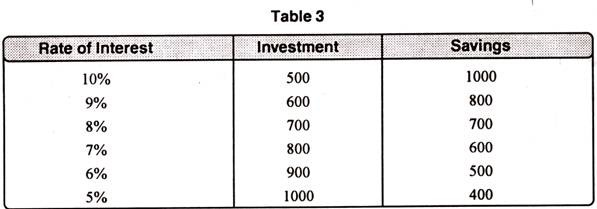The table 3 reveals that equilibrium rate of interest will be determined at a point where demand for and supply of capital are equal. As is clear from the table that equilibrium interest rate 8% is determined because at this level demand for and the supply of capital are equal i.e. Rs. 700 crores.

Now, if the rate of interest increases to 10%, investment is Rs. 500 crores and savings are of Rs. 1000 crores i.e. savings exceed the investment. On the other hand, if the rate of interest falls to 5% investment is Rs. 1000 crores and savings are Rs. 400 crores.

This fact is clearer from the diagram below: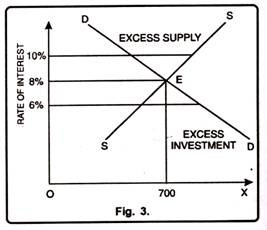In Fig. 3, rate of interest is determined by the intersection of demand and supply curves. Equilibrium is restored at point E which determines rate of interest as 8% and demand and supply of capital as Rs. 700 crores. Now, if the rate of interest increases to 10% supply of savings exceeds the demand for capital i.e. supply is more than demand. This will lead to a fall in interest rate to the level of 8%.

On the other hand, when the interest rate falls to 6%, demand for savings exceeds the supply of savings which will push up the rate of interest to restore an equilibrium rate i.e. 8%. Therefore, rate of interest is in equilibrium only at a point where the demand for capital equals the supply of capital.

### Criticism:

The classical theory of rate of interest has been criticized on the basis of the following shortcomings as discussed below:

#### 1. Indeterminate Theory:

Keynes has maintained that the classical theory is indeterminate in the sense that it fails to determine the interest rate. In this theory, interest is determined by the equality of demand and supply. But the position of savings varies with the income level. Thus, unless we know the income, interest rate cannot be determined.

#### 2. Fixed Level of Income:

Classical theory assumes that the level of income remains constant. But in actual practice income changes with a small change in investment. Thus, it is not correct to assume a fixed level of income.

#### 3. Long Run:

Classical theory determines the interest rate through the interaction of demand and supply of capital in the long run. Keynes pointed out that in the long run we all are dead. Therefore, there was an urgent need of a theory which determines rate of interest in the short-run.

#### 4. Full Employment:

This theory assumes that there is full employment of resources in the economy. But, in reality, unemployment or less than full employment is a general situation. Full employment is only an abnormal case… Thus, this theory does not apply to the present world.

#### 5. Savings and Investment:

Classical economists assume that savings and investment are interring dependent. But actually investment changes, income also changes which leads to a change in savings. Thus, both are interdependent on each other.

#### 6. Ignores Monetary Factors:

Classical theory takes into consideration only the real factors for determining the rate of interest and ignores the monetary factors.